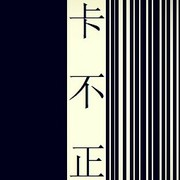[F]_ _ _ _ [C]_ _ _ _ [Am]_ _ _ _ [G]_ _ _ _ [F]_ _ 没想过[C] 有一天[Am] 会想念[G] [F] 以为忘却[C] 不再留恋[Am] 的夏天[G] [F]走 过的街[C] 留下[Am]的 诗[G]篇 [F]我 的眼泪[C] 已干[Am]枯 不[G]见 {start_of_chorus} [F]有一种 遥远[C]的温柔 在你[Am]离开后 悄悄[G] 遗留在我的伤口 [F]有时候 当我[C]想起你 可以[Am]微笑着 难道[G] 你没有把我伤透 {end_of_chorus} {start_of_chorus} [F]荒芜的季节[C] 以为我已告别[Am] 无所谓 [G] 谁该说抱歉 [F]蒸发的世界[C] 你已不在身边[Am] 剩下我 [G] 一个人孤单的冒[F]险 [C] [Am] [G] [F]_ _ _ _ [C]_ _ _ _ [Am]_ _ _ _ [G]_ _ _ _ {end_of_chorus} [F]_ _ 没想过[C] 有一天[Am] 会再见[G] [F] 以为忘却[C] 不再留恋[Am] 的双眼[G] [F]走 过的街[C] 留下[Am]的 诗[G]篇 [F]我 的眼泪[C] 已干[Am]枯 不[G]见 {start_of_chorus} [F]有一种 遥远[C]的温柔 在你[Am]离开后 悄悄[G] 遗留在我的伤口 [F]有时候 当我[C]想起你 可以[Am]微笑着 难道[G] 你没有把我伤透 {end_of_chorus} {start_of_chorus} [F]荒芜的季节[C] 以为我已告别[Am] 无所谓 [G] 谁该说抱歉 [F]蒸发的世界[C] 你已不在身边[Am] 剩下我 [G] 一个人孤单的冒[F]险 [C] [Am] [G] 一个人孤单的想[F]念 {start_of_chorus} {end_of_chorus} {title:蒸发的世界剩下我} {artist:原子邦妮} {author:_wc_wc_}_wc_wc_